ImplicitPlot

Either form of ImplicitPlot will take the options FormatType, ImageSize, TextStyle even though they aren't returned by Options[ImplicitPlot].  ImplicitPlot also takes the options ColorFunction, Compiled, ContourLines, ContourShading and ContourStyle but only when the ContourPlot method is used.  Each of these options except Compiled is demonstrated below.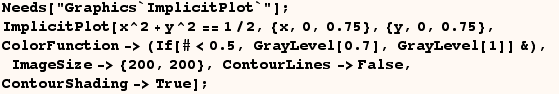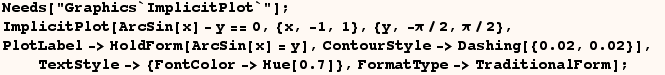The documentation indicates ImplicitPlot uses one of two methods.  In the two cells above the ContourPlot method was used since a range of values for x and y were specified.  When the ContourPlot method is used the ContourStyle option is used to make the curve dotted.  In the next line the Solve method is used since no range is given for the range of (y) values.  Since the Solve method is used the PlotStyle option has to be used to make the curve dotted.  Whether you use PlotStyle or ContourStyle depends on the ImplicitPlot method being used (ContourPlot method, or Solve method).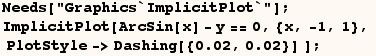Also note the documentation indicates ImplicitPlot uses the same options as plot when the solve method is used.  Actually MaxBend and PlotDivision are plot options, but they aren't recognized by either method of ImplicitPlot.

One should keep in mind that ImplicitPlot uses (AspectRatio→Automatic) by default.  This ensures that the plot of a circle (below) looks like a circle.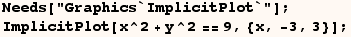However the default option (AspectRatio→Automatic) causes a problem in the next cell.  In this case the range of values on the vertical axis is much larger than the range of values on the horizontal axis.  In this case the default setting for AspectRatio produces a useless graphic.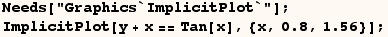Instead the AspectRatio used by most other plot commands (AspectRatio→1/GoldenRatio) produces a much more useful graphic in the next line.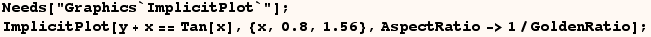In the following line Implicit Plot uses the default options and the curves aren't smooth.  To make the graphs smooth a value for the PlotPoints option has to be specified.  The default setting is PlotPoints->25.  The setting used in the second attempt work better.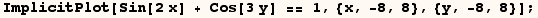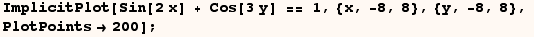Created by Mathematica  (May 16, 2004)

Back to Ted’s Tricks index page